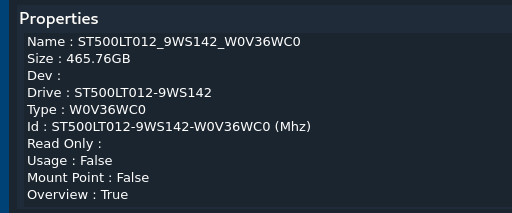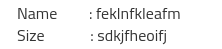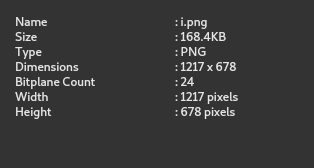# ailing center all text in qstringlist

• here is the stringlist)

i want like thishow can i do that
i tried ").rightJustified()" when adding every string but it is messy and dose not ailing properly.

• Hi
I dont see any difference from the black sample and what you show as example.
Do you mean to pad each string ?

• @mrjj sorry for that.

here is a pic• Hi
Unless you use a monospaced font, its never going to be drawn like that.
Even if you pad Name and Size with spaces, it will not be drawn like that due to varying sizes of the letters.

You could use mono font and use QString split() at ":" and pad the key string
then append with value string.

But often it would just be easier to draw it using tabs or have key and value as seperate string and simply draw it as you want.

Im not sure how you can pad the QStringList and have it shown like that in all cases.

Maybe other have better ideas :)

• QString has nothing to do with rendering the string so it can't be handled in isolation.
From the top of my head, what I would do is:

1. split the list in 2, with one list for the part left of `:` and the other with the right part
2. use `QFontMetrics::width` on each item in the first list to find the longest one
3. use `QFontMetrics::width` on the longest item with a `\t` appended to find the target size
4. append `\t` to the other elements in the list until `QFontMetrics::width` is the same as the target
5. merge the 2 lists back together

• this i came up with .

``````QStringList fStringList(QStringList left, QStringList right, QFont font) {
QFontMetrics *fm = new QFontMetrics(font);
int large = 0;
int index;
for (int i = 0; i < left.count(); i++) {
if (large < fm->width(left.at(i))) {
large = fm->width(left.at(i));
index = i;
}
}

large = large + fm->width('\t');

for (int i = 0; i < left.count(); i++) {
while (large >= fm->width(left.at(i))) {
left.replace(i, QString(left.at(i) + QString('\t')));
}
}

for (int i = 0; i < left.count(); i++) {
left.replace(i, left.at(i) + ": " + right.at(i));
}

return left;
}
``````

using this function

``````fStringList(left, right, ui->detail->font())
``````

output is thisthanks @VRonin